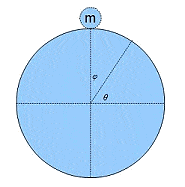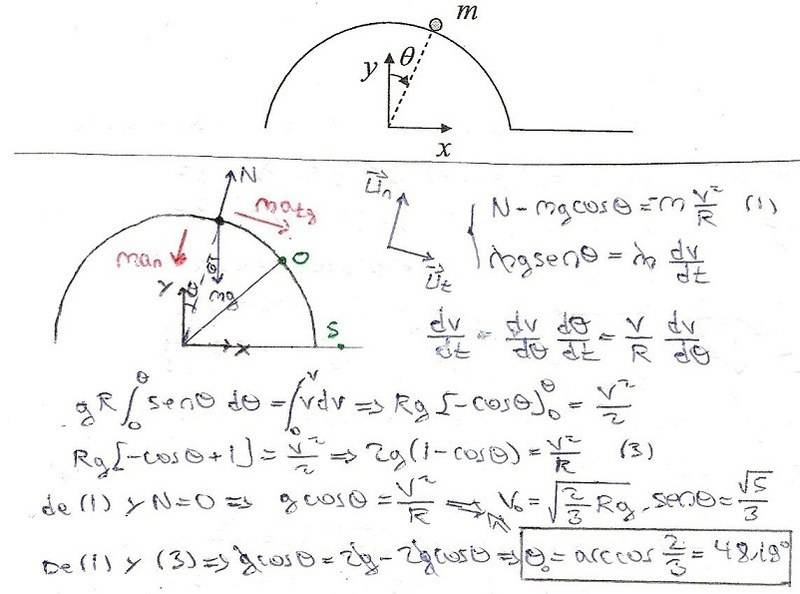# Dynamics of circular motion. Particle on the top of a circle

## Homework Statement

A particle of mass M is on the top of a vertical circle without initial velocity. It starts to fall clockwise.

Find the angle with respect to the origin, where the particle leaves the circle.v=ωXr

## The Attempt at a Solution

I used two unitary vectors..
εr : which direction is the same as the line described by the radio in the separation point.
εθ: perpendicular to εr (the direction of the tangential velocity in the separation point)

r=R er

v=dr/dt = Rder/dt
v=ωXr (counterclockwise, so I changedthe sign to negative)
v=-R(ωXer)

v = -R(-ω) = ωR
α=dω/dt

a = dv/dt = R(α + ω d/dt)

d/dt = -(ωX) = -ωer

a = α R - ω^2 R er

Forces:
Weight, Normal force
Centripetal is a resultant?

F(eθ)==> mgcosθ = αRm
F(er)==> N-mgsenθ= -ω^2Rm

So:

α=dω/dt= (dω/dθ)(dθ/dt)=ωdω/dθ

θ
∫αdθ =
∏/2

ω
∫ωdω
ω0

From F(er) : α= (gcosθ)/R

θ
∫ (gcosθ)/Rdθ = (gsenθ)/R
∏/2

ω
∫ωdω= (ω^2)/2
ω0

ω^2 = (2gsenθ)/R

I come back to the force equations, (with zero normal force) and replace ω^2

And I get nonsenses like 2=1. Did I do something wrong?

Solution: 48,19º (by the teacher)

haruspex
Homework Helper
Gold Member
2020 Award
θ
∫αdθ =
∏/2

ω
∫ωdω
ω0
Not sure exactly where, but you have a sign error. The first integral will be negative, but the second positive.
θ
∫ (gcosθ)/Rdθ = (gsenθ)/R
∏/2
You've not filled in the 'lower' bound correctly after integrating.
ω^2 = (2gsenθ)/R
Could have got this much more quickly and reliably by conservation of energy. Note that the RHS should be 1-sin, not sin.

I found it solved.